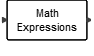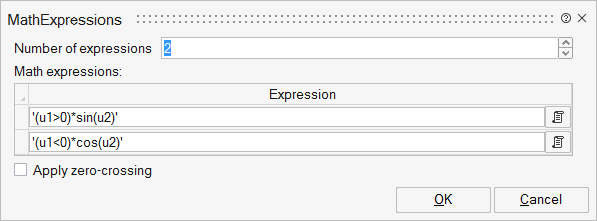# MathExpressions

The output of this block is a vector obtained by evaluating the mathematical expressions specified as block parameters. The expressions are given in terms of variables u1,...un where ui is the ith entry of the unique input vector used by all of the expressions.MathOperations

## Description

The output of this block is a vector obtained by evaluating the mathematical expressions specified as block parameters, each expression corresponds to an element of the resulting vector. The expressions are given in terms of variables u1,...un where ui is the i-th entry of the unique input vector used by all the expressions.

Only double data type is allowed. Supported syntax is listed as following

Arithmetical operator: +, -, *, /, ^

Logical operator: |, &, !, ~

Relational operator: >, <, >=, <=, ==, ~=, <>

Elementary functions: abs, exp ,log, log10, min, max, sign, sqrt, hypot

Trigonometric functions: acos, acosh, asin, asinh, atan, atan2, atanh, cos, cosh, sin, sinh, tan

Rounding functions: ceil, floor, int, round

General math functions: rem power pow

## ParametersNameLabelDescriptionData TypeValid Values

work

Workspace evaluation

used_names

Used names

Hidden parameter used by the block to indicate used environment variables.

nexx

Number of expressions

Number of expressions that should be evaluated.

Number

Exp

Math expressions:

Mathematical expressions that should be evaluated based on the inputs.

Structure

Exp/exx

Expression

Cell of strings

zc

Apply zero-crossing

Enables the detection of zero crossings.

Scalar

0
1

## Ports

NameTypeDescriptionIO TypeNumber

Port 1

explicit

output

1

Port 2

explicit

input

1

NameValueDescription

always active

no

direct-feedthrough

yes

zero-crossing

depends on block parameters

mode

depends on block parameters

continuous-time state

no

discrete-time state

no